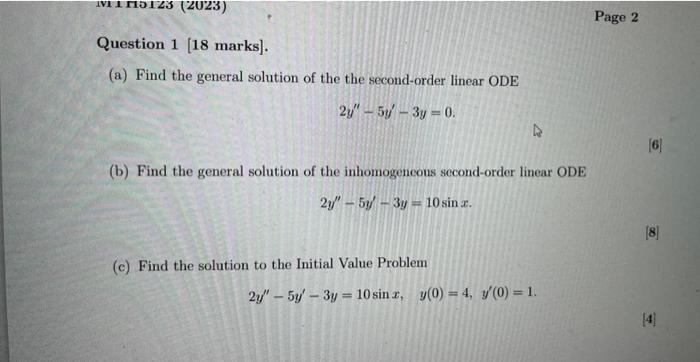Home / Expert Answers / Calculus / a-find-the-general-solution-of-the-the-second-order-linear-ode-2-y-prime-prime-5-y-prime-pa397

# (Solved): (a) Find the general solution of the the second-order linear ODE $2 y^{\prime \prime}-5 y^{\prime ...(a) Find the general solution of the the second-order linear ODE \[ 2 y^{\prime \prime}-5 y^{\prime}-3 y=0$ (b) Find the general solution of the inhomogeneous sccond-order linear ODE $2 y^{\prime \prime}-5 y^{\prime}-3 y=10 \sin x$ (c) Find the solution to the Initial Value Problem $2 y^{\prime \prime}-5 y^{\prime}-3 y=10 \sin x, \quad y(0)=4, y^{\prime}(0)=1 .$

We have an Answer from Expert